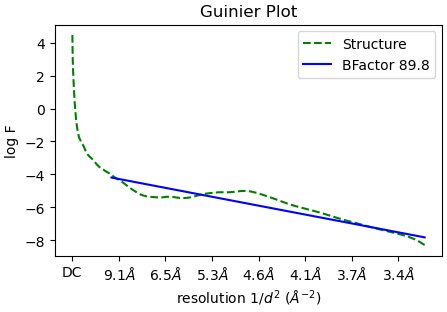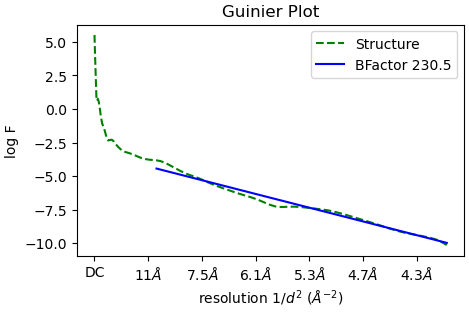# Estimated Bfactor differ by a factor of two compared to RELION

Hi,

I run a ‘Reconstruct only’ job and got half A(J**_volume_map_half_A.mrc), half B(J**_volume_map_half_B.mrc), and a mask(J**_volume_mask_fsc.mrc).

In the ‘Reconstruct only’ job, the estimated Bfactor is -89.8:But if I use RELION for post-processing with the same half maps and mask as input, the estimated B factor differs by a factor of two compared to CryoSPARC：

`relion_postprocess --i J214_volume_map_half_A.mrc --i2 J214_volume_map_half_B.mrc --o relion_sharp --angpix 0.644 --mask J214_volume_mask_fsc.mrc --auto_bfac true`

It reports:

``````== Reading input half-reconstructions:
+ half1-map:                     J214_volume_map_half_A.mrc
+ half2-map:                     J214_volume_map_half_B.mrc
+ --mtf_angpix was not provided, assuming pixel size in raw micrographs is the same as in particles, 0.644 A/px.
== Using a user-provided mask ...
+ fraction f (solvent mask based): 35.6179
+ molecular weight inside protein mask: 259965
+ randomize phases beyond:       14.4256 Angstroms
== Applying sqrt(2*FSC/(FSC+1)) weighting (as in Rosenthal & Henderson, 2003) ...
== Fitting straight line through Guinier plot to find B-factor ...
+ fit from resolution:           10
+ fit until resolution:          0
+ slope of fit:                  -11.6472
+ intercept of fit:              -10.8523
+ correlation of fit:            0.792205
+ apply b-factor of:             -46.5887
== Writing output files ...
+ Processed map:                 relion_sharp.mrc
+ FINAL RESOLUTION:              3.24171
``````

With the same half maps and mask, RELION apply b-factor of -46.5887, while CryoSPARC apply a b-factor of -89.8.

I wonder why does estimated Bfactor in CryoSPARC differ by a factor of two compared to RELION. (My cryoSPARC is v4.1.0)

BTW, in the source code of RELION, I can see that RELION divide high frequency part by e^{-0.25 b x^2}:

``````void applyBFactorToMap(MultidimArray<Complex > &FT, int ori_size, RFLOAT bfactor, RFLOAT angpix)
{
FOR_ALL_ELEMENTS_IN_FFTW_TRANSFORM(FT)
{
int r2 = kp * kp + ip * ip + jp * jp;
RFLOAT res = sqrt((RFLOAT)r2)/(ori_size * angpix); // get resolution in 1/Angstrom
if (res <= 1. / (angpix * 2.) ) // Apply B-factor sharpening until Nyquist, then low-pass filter later on (with a soft edge)
{
DIRECT_A3D_ELEM(FT, k, i, j) *= exp( -(bfactor / 4.)  * res * res);
}
else
{
DIRECT_A3D_ELEM(FT, k, i, j) = 0.;
}
}
}
``````

I wonder how whether the coefficient for B-factor sharpening in CryoSPARC is the same as that used by RELION.

Thank you very much!

I also run a ‘Homogeneous Refinement’ job and got half maps and mask in final iteration rounds. Denote final round as x, with x typically ranging from 6 to 8:

half A: J**_00x_volume_map_half_A.mrc,
half B: J**_00x_volume_map_half_B.mrc,

For this homogeneous refinement job, CryoSPARC estimate b factor of -230.5.I also use RELION for post-processing with the same half maps and mask as input,

`relion_postprocess --i J170_007_volume_map_half_A.mrc --i2 J170_007_volume_map_half_B.mrc --o relion_sharp --angpix 1.3099979 --mask J170_007_volume_mask_fsc.mrc --auto_bfac true`

RELION apply b-factor of -152.613;

If I use J170_007_volume_mask_refine.mrc instead of J170_007_volume_mask_fsc.mrc, RELION apply b-factor of -156.576, similar as previous one in RELION.

This time, for refinement job, estimated Bfactor in CryoSPARC still differs from that in RELION, but the difference is no longer a factor of two.

This suggests that the discrepancy in the estimated B-factor between CryoSPARC and RELION may be related to the specific job used in CryoSPARC.

At the end of refinement, cryoSPARC performs auto-tightening of the refinement mask to generate a mask with suffix `mask_fsc_auto`. Does that change the `relion_postprocess` calculations?

Cheers,
Yang

Thank you!

For the refinement job above, I also tried to use `mask_fsc_auto` as the input of relion_postprocess:

`relion_postprocess --i J170_007_volume_map_half_A.mrc --i2 J170_007_volume_map_half_B.mrc --o relion_sharp --angpix 1.3099979 --mask J170_007_volume_mask_fsc_auto.mrc --auto_bfac true`

Now I get:

``````== Reading input half-reconstructions:
+ half1-map:                     J170_007_volume_map_half_A.mrc
+ half2-map:                     J170_007_volume_map_half_B.mrc
+ --mtf_angpix was not provided, assuming pixel size in raw micrographs is the same as in particles, 1.31 A/px.
== Using a user-provided mask ...
+ fraction f (solvent mask based): 50.7525
+ molecular weight inside protein mask: 286528
+ randomize phases beyond:       9.5817 Angstroms
== Applying sqrt(2*FSC/(FSC+1)) weighting (as in Rosenthal & Henderson, 2003) ...
== Fitting straight line through Guinier plot to find B-factor ...
+ fit from resolution:           10
+ fit until resolution:          0
+ slope of fit:                  -32.9301
+ intercept of fit:              -10.3171
+ correlation of fit:            0.926371
+ apply b-factor of:             -131.72
== Writing output files ...
+ Processed map:                 relion_sharp.mrc
+ FINAL RESOLUTION:              4.14024
``````

Estimated b factor in RELION is -131.72 and Estimated b factor in CryoSPARC is -230.5.

I would wait for one of the team to respond, but looking through my logs, `relion_postprocess` and cryoSPARC arrive coincidentally at very similar B-factors for our data.

RELION calculates the B-factor by multiplying the slope of the Guinier plot fit by a factor of 4. Eyeballing the Guinier plots that cryoSPARC makes, this seems to hold as well, after a ~2.3 conversion factor between the natural logarithm (RELION) and log base 10 (cryoSPARC). I suppose the difference must lie in the fitting of the slope–perhaps in the data-limits? Does one fit to Nyquist whereas the other limits to a frequency where FSC=0.143?

Cheers,
Yang

Hey @Joey, from the RELION log, two key differences are that CS fits from 10A to 0.143 FSC resolution instead of 10A to Nyquist resolution, and doesn’t perform FSC weighting on the input volume. I haven’t looked closely at the RELION code, but this may explain the discrepancy.

Also, for masks, Homogeneous Refinement uses the one saved in the `mask.mask_refine` output for B-factor estimation, and Reconstruct Only uses the input mask.

1 Like

Thank you very much for your help!

Do you mean that the b factor estimation in CryoSPARC is performed on the map without FSC weighting?

I also tried to perform `relion_postprocess` using mask_refine.mrc and fits from 10A to 0.143 FSC resolution.

`relion_postprocess --i J175_007_volume_map_half_A.mrc --i2 J175_007_volume_map_half_B.mrc --o relion_sharp --angpix 1.3099979 --mask J175_007_volume_mask_refine.mrc --auto_bfac true --autob_lowres 10 --autob_highres 4.1`

Estimated b factor in CryoSPARC is -232.0 and Estimated b factor in RELION is -131.579.

RELION log is as follows:

``````== Reading input half-reconstructions:
+ half1-map:                     J175_007_volume_map_half_A.mrc
+ half2-map:                     J175_007_volume_map_half_B.mrc
+ --mtf_angpix was not provided, assuming pixel size in raw micrographs is the same as in particles, 1.31 A/px.
== Using a user-provided mask ...
+ fraction f (solvent mask based): 22.6343
+ molecular weight inside protein mask: 642475
+ randomize phases beyond:       10.48 Angstroms
== Applying sqrt(2*FSC/(FSC+1)) weighting (as in Rosenthal & Henderson, 2003) ...
== Fitting straight line through Guinier plot to find B-factor ...
+ fit from resolution:           10
+ fit until resolution:          4.1
+ slope of fit:                  -32.8948
+ intercept of fit:              -10.415
+ correlation of fit:            0.894469
+ apply b-factor of:             -131.579
== Writing output files ...
+ Processed map:                 relion_sharp.mrc
+ FINAL RESOLUTION:              4.19199
``````

If I add `--skip_fsc_weighting` to `relion_postprocess` above:

`relion_postprocess --i J175_007_volume_map_half_A.mrc --i2 J175_007_volume_map_half_B.mrc --o relion_sharp --angpix 1.3099979 --mask J175_007_volume_mask_refine.mrc --auto_bfac true --autob_lowres 10 --autob_highres 4.1 --skip_fsc_weighting`

Estimated B factor in RELION is even smaller(-90.24), still different with CSPARC(-232.0)

``````== Reading input half-reconstructions:
+ half1-map:                     J175_007_volume_map_half_A.mrc
+ half2-map:                     J175_007_volume_map_half_B.mrc
+ --mtf_angpix was not provided, assuming pixel size in raw micrographs is the same as in particles, 1.31 A/px.
== Using a user-provided mask ...
+ fraction f (solvent mask based): 22.6343
+ molecular weight inside protein mask: 642475
+ randomize phases beyond:       10.48 Angstroms
== Fitting straight line through Guinier plot to find B-factor ...
+ fit from resolution:           10
+ fit until resolution:          4.1
+ slope of fit:                  -22.5624
+ intercept of fit:              -10.5042
+ correlation of fit:            0.743929
+ apply b-factor of:             -90.2495
== Writing output files ...
+ Processed map:                 relion_sharp.mrc
+ FINAL RESOLUTION:              4.19199
``````

In the source code of RELION, estimated b factor is four times the slope in GuinierPlot:

``````		fitStraightLine(guinierweighted, global_slope, global_intercept, global_corr_coeff);
global_bfactor = 4. * global_slope;
``````

I wonder if the b factor in CSPARC is the same meaning as RELION.

Thank you very much. Any advice would help!

To estimate the B-factor, we just need to know:

1. the map used for the Guiner plot(thus for B-factor estimating), including the processing applied to the half maps.

2. for the slope fit to the Guiner plot, the B-factor estimated by CryoSPARC is how many times the slope.

I want to report the different B-factors for different datasets in my work, so I want to understand why the B-factor differs between RELION and CryoSPARC for my datasets.

Thank you very much!

If you run the b-factor calculation script from RELION (which does a lot of reconstructions with different particle counts to calculate it) you’ll get a different result again…

The important word is “estimated”.I understand that the b factor is an estimated value, and different software may have varying degrees of numerical error, but the current difference of 2-3 times seems unlikely to be solely attributed to numerical error. I am worried that reporting such a large difference in the b factor in my paper may be questioned by reviewer.

I prefer to go by the b-factor calculation script in RELION, but it takes time to run.

Hi @Joey, sorry for the delayed response. Turns out the difference is that RELION uses the unmasked volume for B-factor estimation, while CS uses the masked volume. You can see in RELION’s code that it performs masking for FSC and phase randomization, then re-imports the original half maps before B-factor estimation. If you’re skipping FSC weighting, the input mask has no impact on the estimated B-factor.

For a dataset which originally had a RELION B-factor of -6.85, I applied the mask in `mask_refine` to the half-maps in Chimera, and then reran `relion_postprocess` with `--autob_highres` at FSC resolution and `--skip_fsc_weighting`:

``````relion_postprocess --i J454_half_map_A_masked.mrc --i2 J454_half_map_B_masked.mrc --angpix 0.8320 --o relion_sharp_3 --auto_bfac true --autob_lowres 10 --autob_highres 4.13 --skip_fsc_weighting
+ --mtf_angpix was not provided, assuming pixel size in raw micrographs is the same as in particles, 0.832 A/px.
== Not performing any masking ...
+ randomize phases beyond:       10.6496 Angstroms
== Fitting straight line through Guinier plot to find B-factor ...
+ fit from resolution:           10
+ fit until resolution:          4.13
+ slope of fit:                  -26.4981
+ intercept of fit:              -11.9315
+ correlation of fit:            0.986095
+ apply b-factor of:             -105.992
== Writing output files ...
+ Processed map:                 relion_sharp_3.mrc
The values still won’t be identical because the order of operations in the two implementations are slightly different, but they should definitely not be off by multiples of each other. As of right now there’s no way to enforce masking in `relion_postprocess`, and no way to turn off masking in CS B-factor estimation, but we’ll consider adding the option in a future update